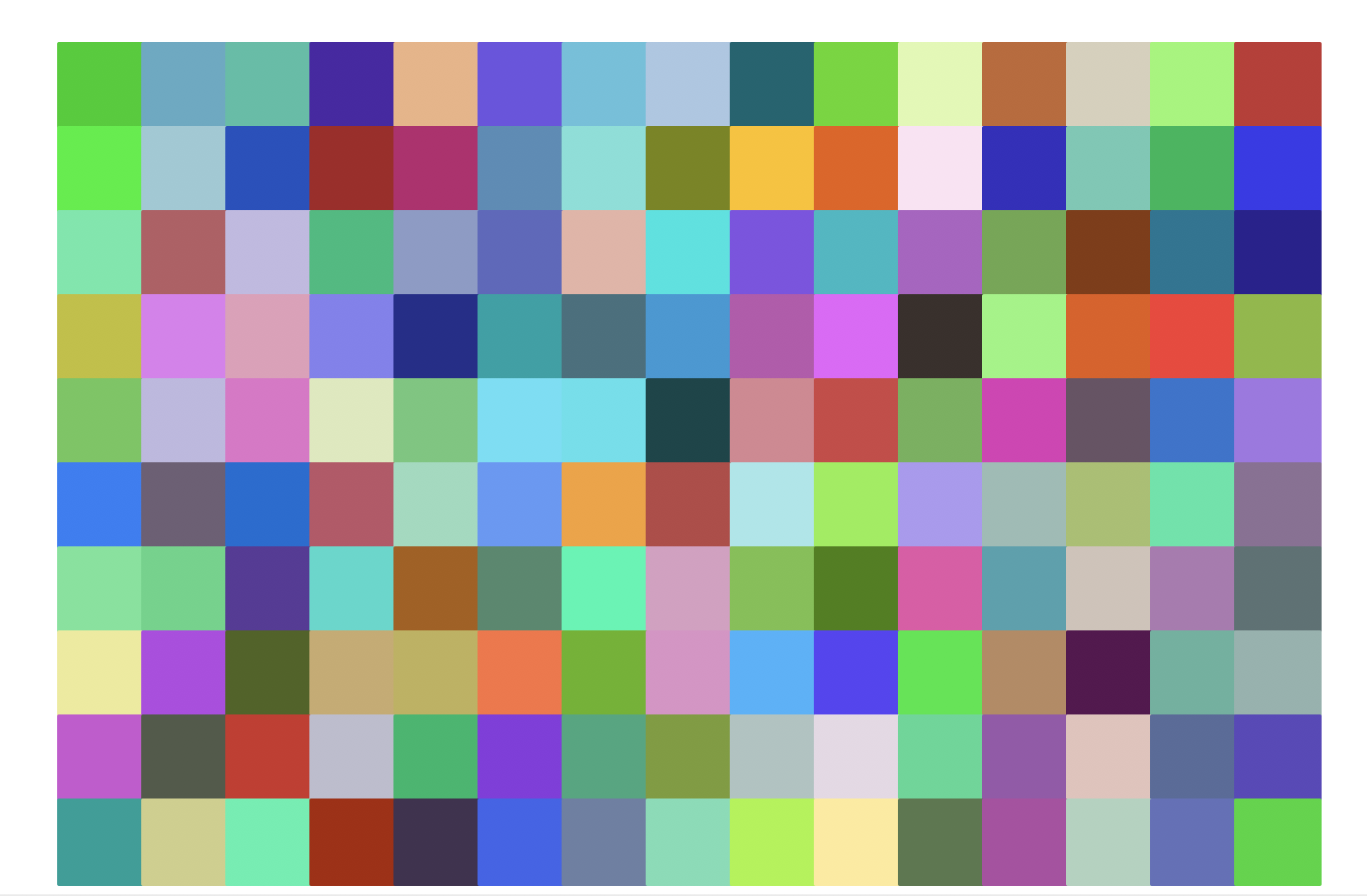# Lab 5, Part 2: Turtle Graphics with For Loops

This part of today's lab should be completed in the provided `lab05/grids.py` file.

## Task A. `randomSquares`

In this task, you'll complete a function called `randomSquares` that draws random square on the canvas. Below two different invocations are shown on the same canvas:

``````randomSquares(10, 50, 'red')  # 10 red randomly-positioned squares of size 50
randomSquares(20, 10, 'cyan') # 20 cyan randomly-positioned squares of size 10``````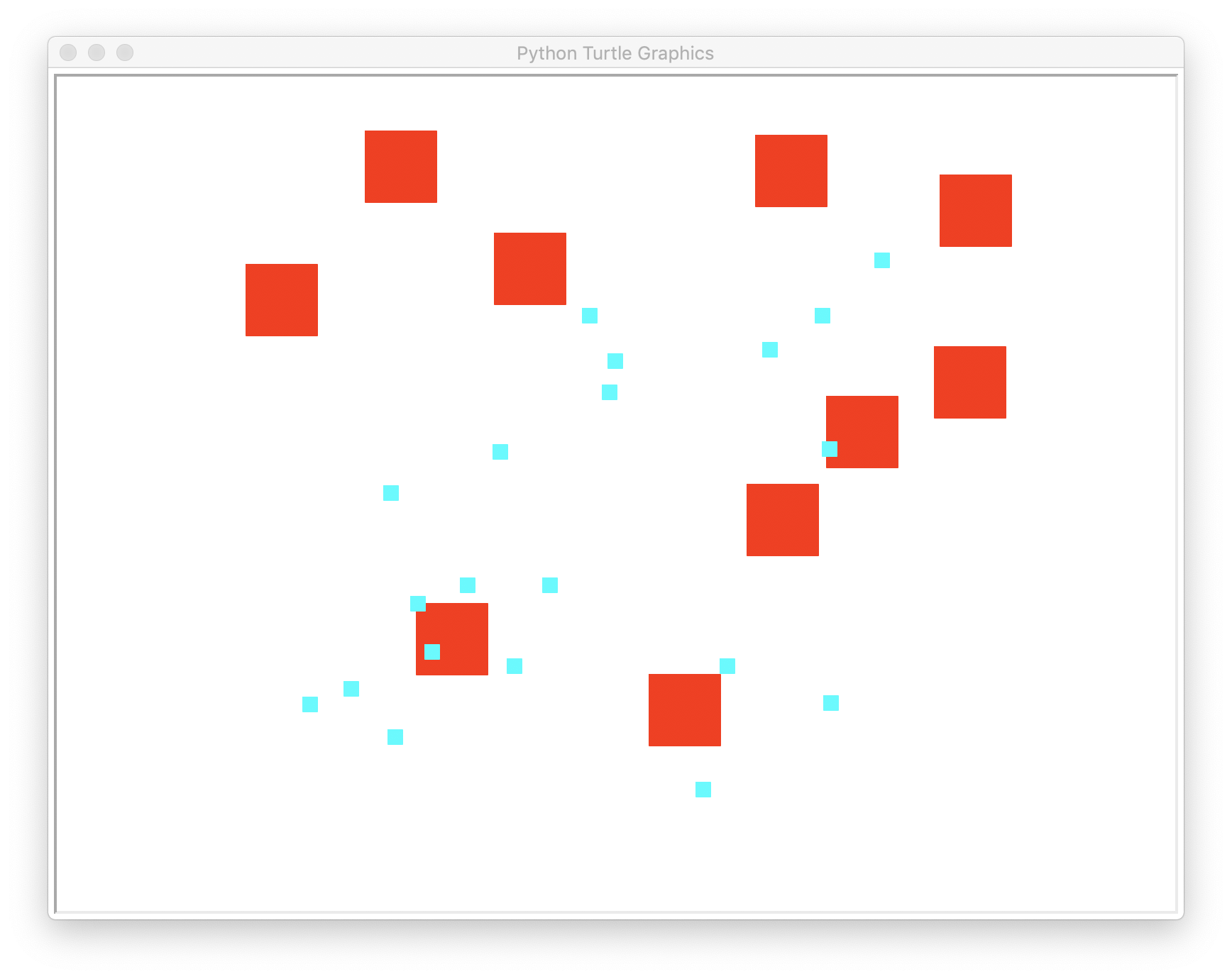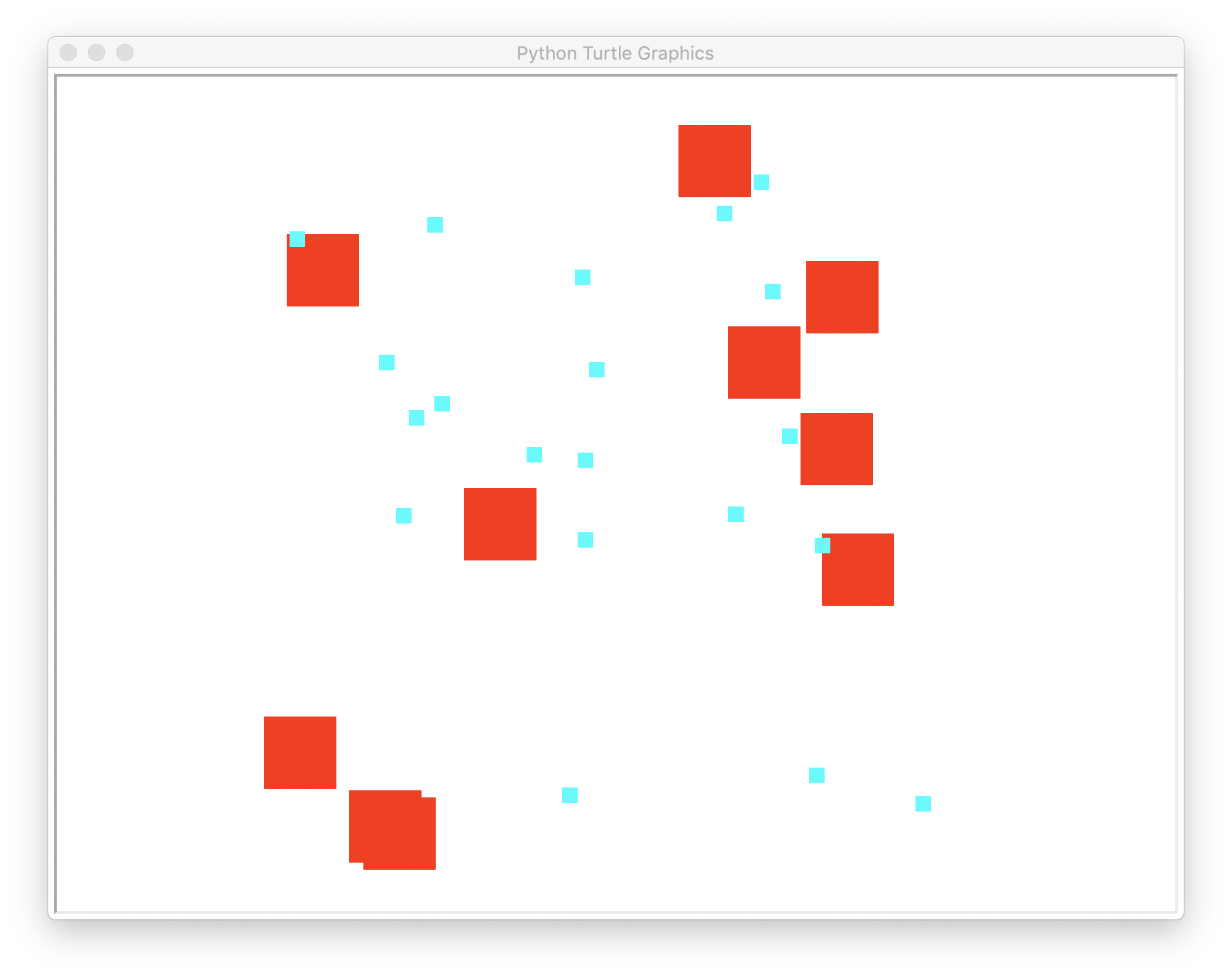Your `randomSquares` function should take the following 3 parameters:

1. `number` of squares in the Layer
2. `size` of the individual squares
3. `color` of the squares

Each time through the loop, one square is drawn. The turtle is repositioned before drawing each square at a randomly generated location (the x coordinate of the center point is a random integer between -400 and 400, and the same is true for the y coordinate of the center point).

Hint: use `random.randint()`. Here are two `randint` refresher links: last week's lab with the coin flip & general python documentation pages for random numbers.

Develop your code in baby steps. Write your function, at first, to simply produce the right number of squares. After that works, then work on placing them in random locations.

Tips:

1. Each time through the loop, you will draw another square
2. The function is not fruitful; it merely draws
3. Since the points are random, your results will differ from what is depicted above

Here is how you can test your `randomSquares` function:

``````if __name__ == "__main__":

#                         replace these with actual values
randomSquares(HOW_MANY, SIZE, COLOR)
``````

Note that the above testing code should be placed in the `__name__ == "__main__"` conditional found in `lab05/grids.py`; you can assume all upcoming test invocations should also go in this main conditional. Ref: Disabling test work)

## Task B. `makeRow`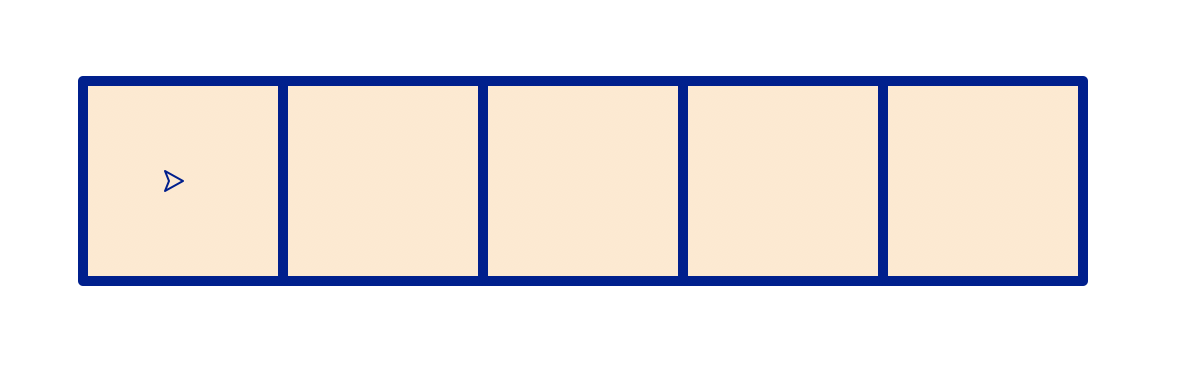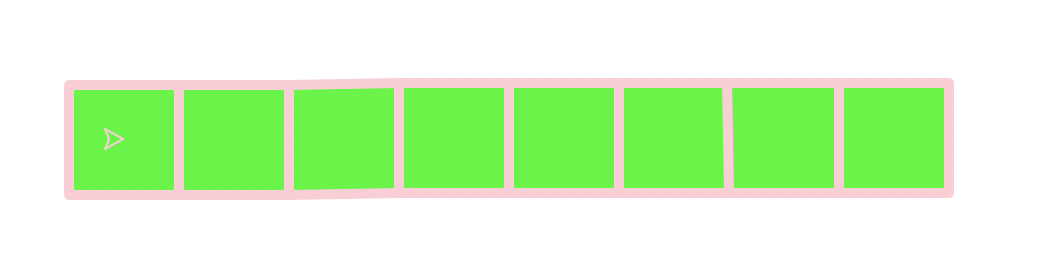In this task, you'll complete a function called `makeRow` that draws a row of squares.

This function should take the following 4 parameters:

1. `number` of squares in the row
2. `size` of the individual squares
3. `color` of the outline of the squares
4. `color` of the fill of the squares

The row is produced by adding one new square each time through the loop.

Tips:

1. Each time through the loop, you will add draw another square
2. Each successive square's center point shifts `size` to the right
3. The function is not fruitful; it merely draws
4. The function maintains a heading and position invariant (so the turtle ends up where it started when the function is complete)

Here is how you can test your `makeRow` function:

``````if __name__ == "__main__":

makeRow(5, 100, 'navy', 'bisque')
makeRow(8, 55, 'pink', 'green')
``````

## Task C. `makeOverlapRow`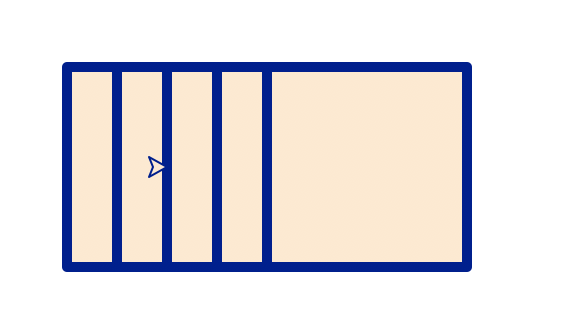Create a new function called `makeOverlapRow` that draws a row of overlapping squares.

This function takes 4 parameters:

1. the `number` of squares
2. the `size` of the square
3. the `color` of the outline of the square
4. the `color` of the interior of the square

Tips:

1. Each time through the loop, you will add draw another square
2. Each successive square's center point shifts `size`/4 to the right
3. The function is not fruitful; it merely draws
4. The function maintains a heading and position invariant (so the turtle ends up where it started when the function is complete)

Starting testing code (needs to be completed):

``````# [your code here] # Draw the overlapping row
``````

## Task D. `makeTwistySquares(number, size, angle)`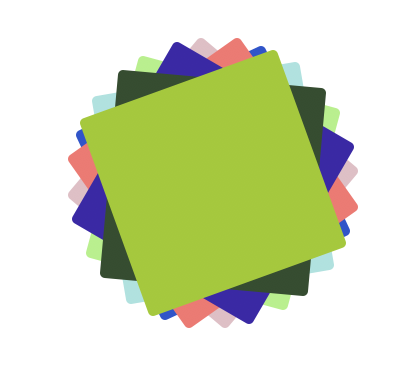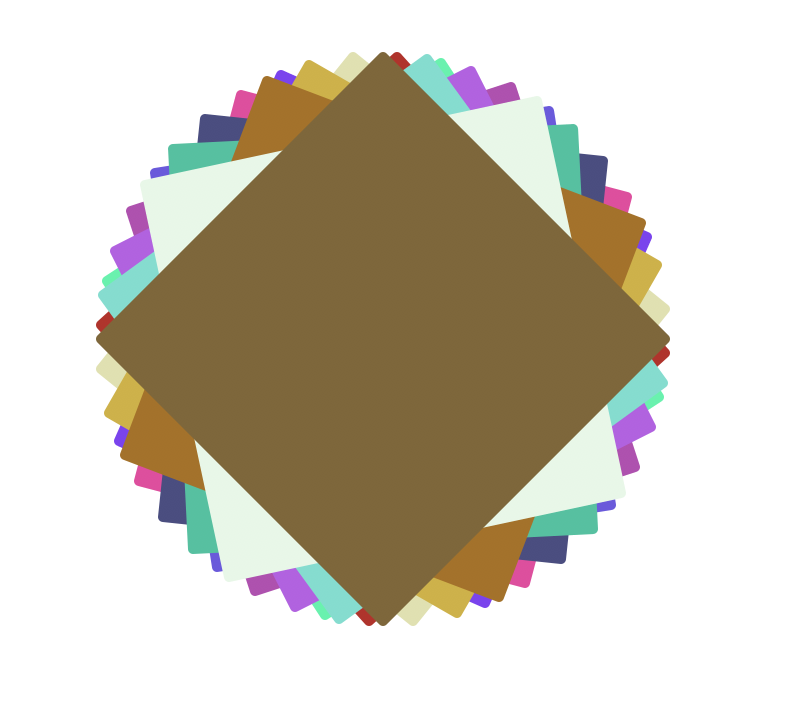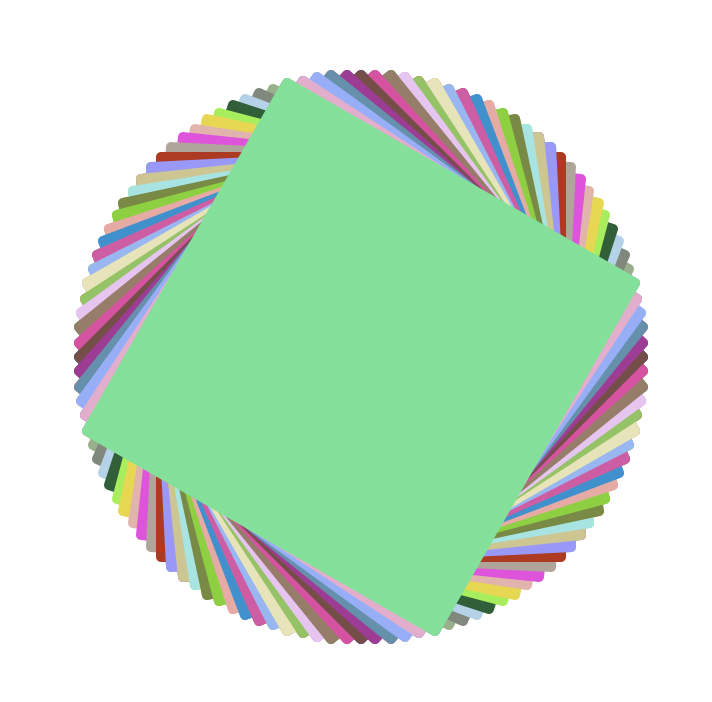Create a new function called `makeTwistySquares` that draws a set of overlapping rotated squares of varying colors.

This function should take the following 3 parameters:

1. `number` of squares
2. `size` of the square
3. `angle` of rotation

Like the previous function, colors should randomly be generated for each square.

Tips:

1. Each time through the loop, you will draw another square
2. Each successive square rotates `angle` degrees more than its predecessor
3. Use the provided `randomTurtleColor()` function to generate a random color for each square drawn, or use one of the random color functions found in `turtleBeads.py`, like `randomPastelColor` or `randomWarmColor`.
4. The function is not fruitful; it merely draws
5. The function maintains a heading and position invariant (so the turtle ends up where it started when the function is complete)

Testing code (the images above were generated with the following invocations, each on a new canvas):

``````   makeTwistySquares(8, 100, 25)
makeTwistySquares(15, 200, 33)
makeTwistySquares(50, 200, 3)
``````

## Task E. `makeRotatedRow`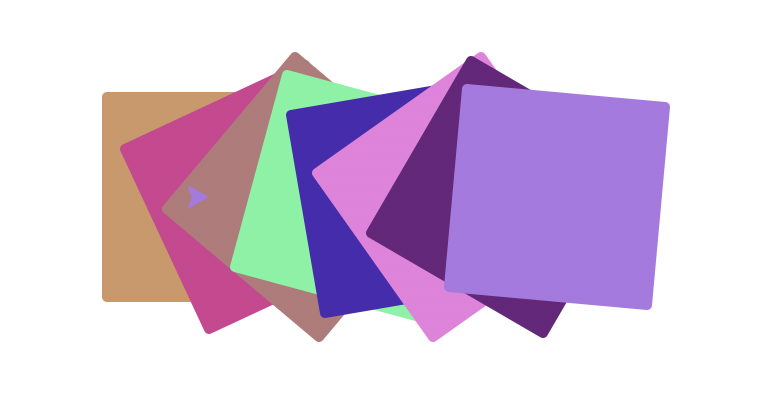Create a new function called `makeRotatedRow` that draws a row of rotated squares of varying colors.

This function takes the following 3 parameters:

1. `number` of squares
2. `size` of squares
3. `angle` of rotation

Note there is no color parameter; each square's color should be randomly generated using the provided function `randomTurtleColor()`, or one of the random color functions from `turtleBeads`; example usage:

``````# sets variable randomColor to store a randomly generated color
randomColor = randomTurtleColor()
# picks from a specific set of warm colors (see turtleBeasd.py)
randomColor = randomWarmColor()``````

(randomTurtleColor works by randomly generating three integers between [0, 1] that are the Red, Green and Blue values, respectively. You can read more about RGB colors here)

Tips:

1. Each time through the loop you will draw another square
2. Each successive square's center point shifts `size`/4 to the right
3. Each successive square rotates `angle` degrees more than its predecessor
4. The function is not fruitful; it merely draws
5. The function maintains a heading and position invariant (so the turtle ends up where it started when the function is complete)

## Task F [challenge problem]. `makeFlower`

Create a new function called `makeGrid` that draws a set of squares arranged in a circle-like shape, with each corner touching the corner of the two adjacent squares. This function takes the following parameters:

1. Number of squares (`petals`)
2. `size` of each square
3. `color` of all the squares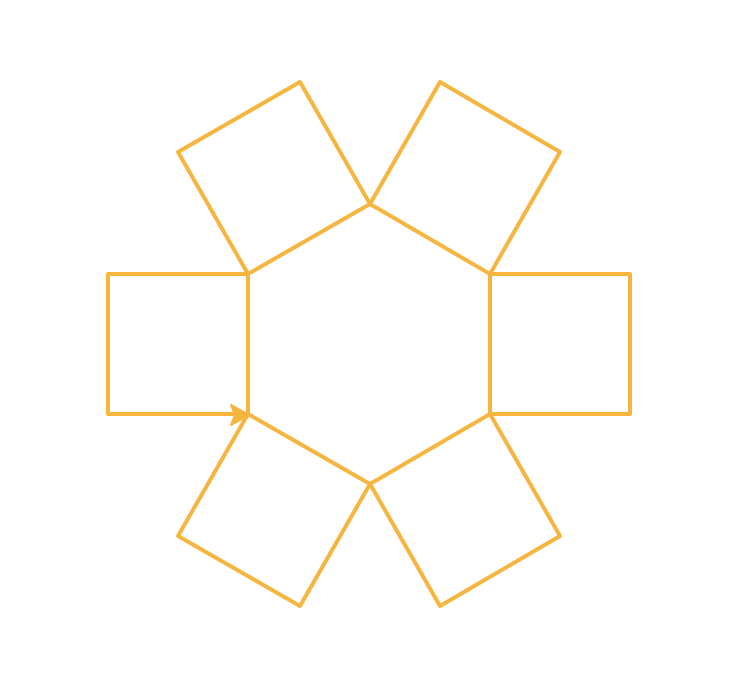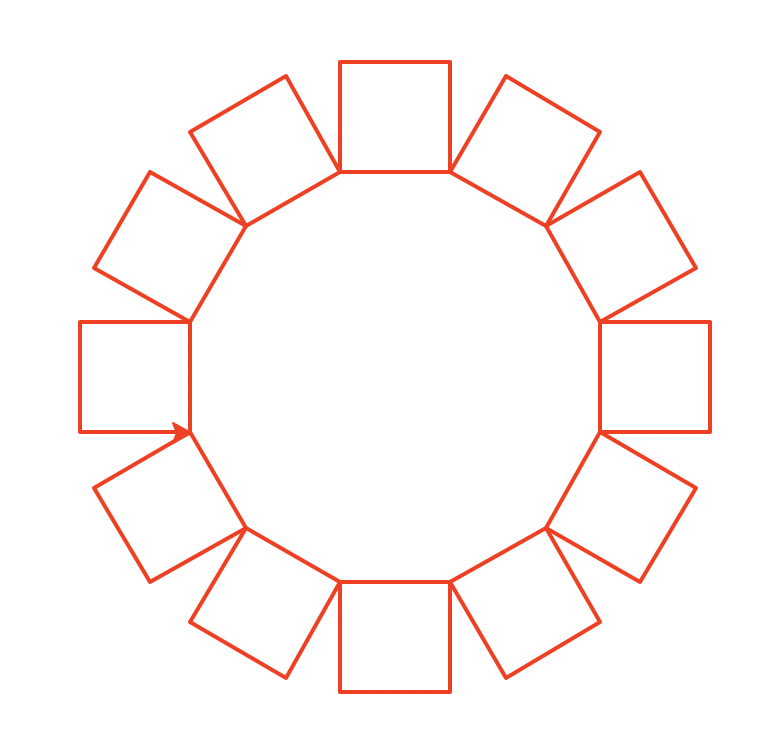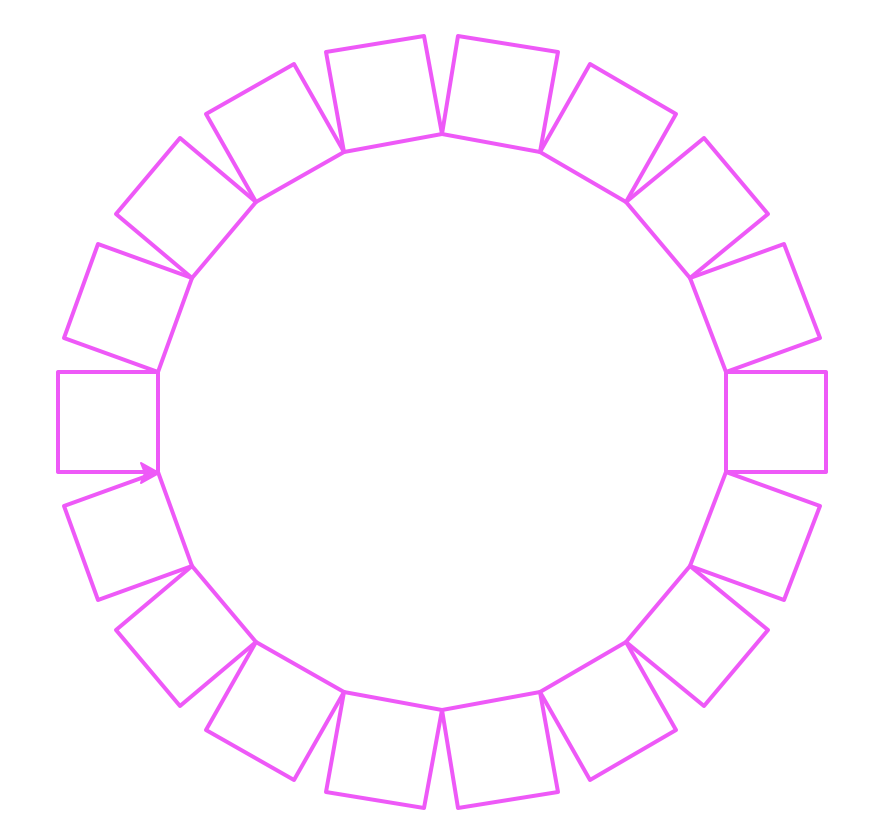Tips:

1. Each time through the loop, you will draw another square
2. You can divide `360/number of squares` to determine the angle of rotation
3. The function is not fruitful; it merely draws
4. The function maintains a heading and position invariant (so the turtle ends up where it started when the function is complete). In this case, the turtle starts and finishes at the lower right corner of the leftmost flower "petal". If you look carefully, you can see the turtle in each of the images above.

Examples:

``````makeFlower(6, 70, 'orange')
makeFlower(12, 55, 'red')
makeFlower(18, 50, 'magenta')``````

## Task G. `makeGrid`

Create a new function called `makeGrid` that draws a set of squares arranged in a grid. This function takes the following parameters:

1. `rows` in the grid
2. `columns` in the grid
3. The `size` of each square
4. `color1` outer square color
5. `color2` fill square color

Examples:

``makeGrid(8, 3, 50, 'orange', 'grey')``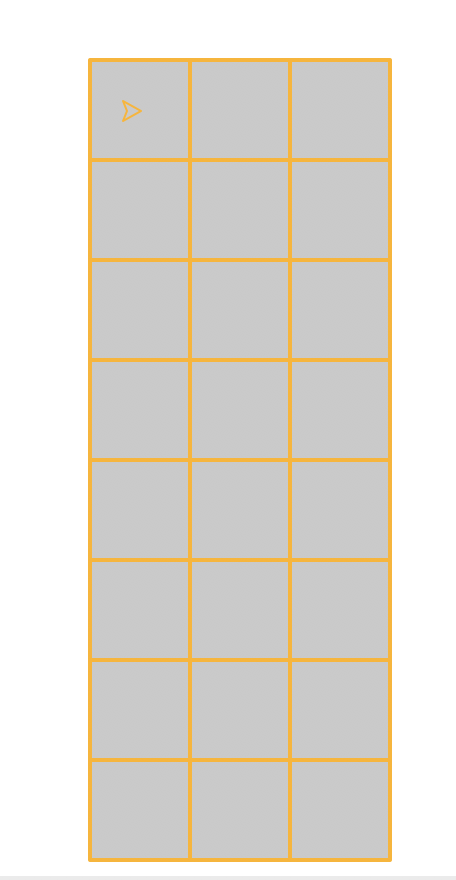``makeGrid(4, 10, 50, 'gray', 'black')``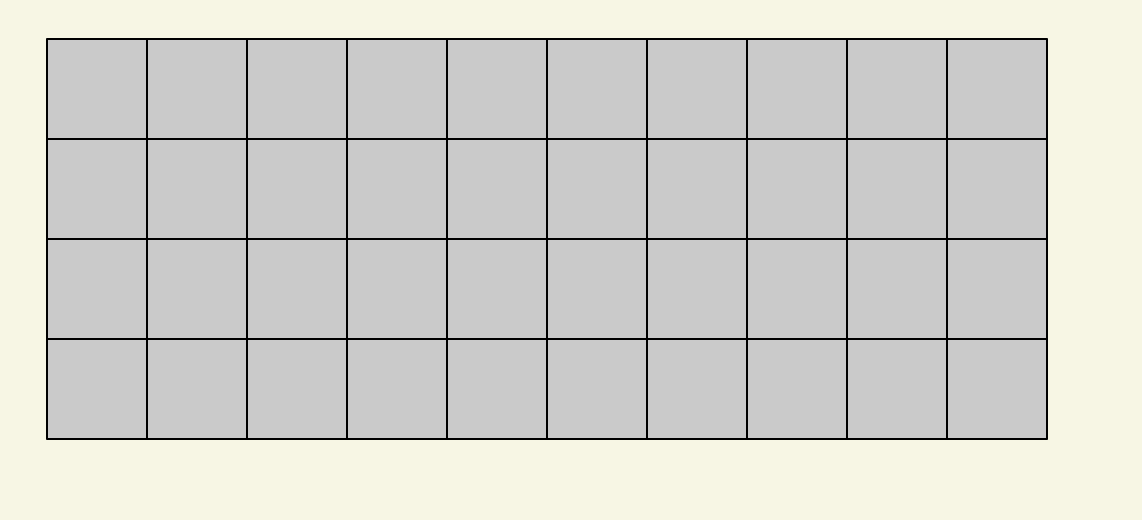``makeGrid(2, 15, 30, 'black', 'orange')``

<img src="orange.png" style="width:300px" alt="A 15-column 2-row grid with orange squares."

The grids can be large, so make sure to start with a fresh canvas for each one.

Tips:

1. You can use a nested loop and draw squares, or you can use a loop that invokes `makeRow`
2. The function is not fruitful; it merely draws
3. Make sure you test your function on a variety of different sized grids

## Task H. `makeRowColorGrid`

Create a new function named `makeRowColorGrid` that draws squares arranged in a grid, where each row of squares is a random color.

This function may, but does not need to, use the `makeRow` function you've already created.

This function should take the following 3 parameters:

1. Number of `rows`
2. Number of `columns`
3. `size` of the individual squares

Example

``makeRowColorGrid(8, 3, 50)``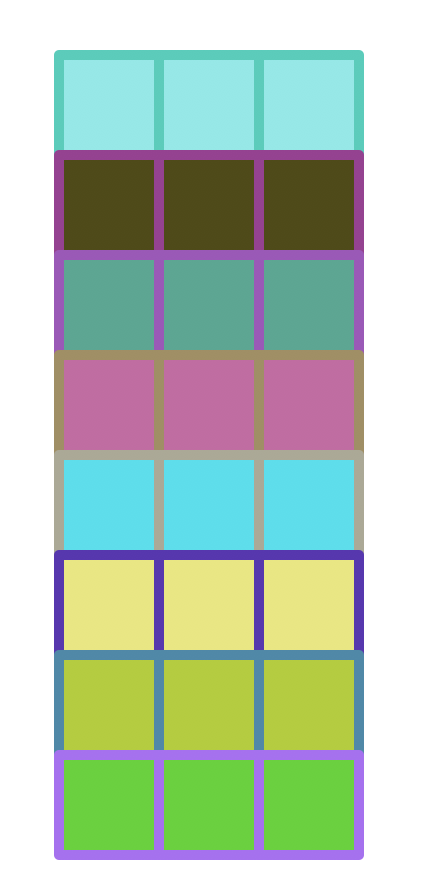## Task I. `makeRandomColorGrid`

Create a new function named `makeRandomColorGrid` that draws squares arranged in a grid, where each row of squares is a random color.

This function should take the following 3 parameters:

1. Number of `rows`
2. Number of `columns`
3. `size` of the individual squares

Each square has a randomly assigned color (use the provided `randomTurtleColor()` function).

``makeRandomColorGrid(10, 15, 50)``